# Civil Engineering - Applied Mechanics

### Exercise :: Applied Mechanics - Section 1

21.

If the tension in a cable supporting a lift moving upwards is twice the tension when the lift is movng downwards, the acceleration of the lift, is

 A.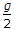B.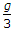C.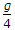D.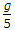Explanation:

No answer description available for this question. Let us discuss.

22.

One Newton force, is

 A. 103 dynes B. 104 dynes C. 105 dynes D. 106 dynes E. 107 dynes.

Explanation:

No answer description available for this question. Let us discuss.

23.

The masses of two balls are in the ratio of 2 : 1 and their respective velocities are in the ratio of 1 : 2 but in opposite direction before impact. If the coefficient of restitution is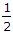, the velocities of separation of the balls will be equal to

 A. original velocity in the same direction B. half the original velocity in the same direction C. half the original velocity in the opposite direction D. original velocity in the opposite direction

Explanation:

No answer description available for this question. Let us discuss.

24.

The angle which an inclined surface makes with the horiontal when a body placed on it is on the point of moving down, is called

 A. angle of repose B. angle of friction C. angle of inclination D. none of these.

Explanation:

No answer description available for this question. Let us discuss.

25.

Work may be defined as

 A. force x distance B. force x velocity C. force x acceleration D. none of these.